﻿ Tunable Photonic Band Gap in a One-Dimensional Lattice Substituted Multiferroic - Dielectric Photonic Crystals in Near Infrared Region

### Tunable Photonic Band Gap in a One-Dimensional Lattice Substituted Multiferroic - Dielectric Photoni...

Sanjay SrivastavaOPEN ACCESSPEER-REVIEWED

## Tunable Photonic Band Gap in a One-Dimensional Lattice Substituted Multiferroic - Dielectric Photonic Crystals in Near Infrared Region

Sanjay SrivastavaDepartment of Materials Science and Metallurgical Engineering, Maulana Azad National Institute of Technology, Bhopal, India

### Abstract

This document gives formatting instructions for authors preparing papers for publication in the journal. Authors are encouraged to prepare manuscripts directly using this template. This template demonstrates format requirements for the JournalIn order to investigate band gap tunability in polar oxides, the photonic band gap of antiferromagnetic-dielectric binary photonic crystal can be significantly enlarged by the substitution of the lattice atoms with other suitable atoms which forms a solid solution with the parent lattice. In this paper we measured the optical properties of a series of Bi (Fe1−xMnx) O3 thin films. Two substrates with different orientation of the crystal plane were selected which were also acting as dielectric materials in multilayer photonic crystals. The absorption response of the mixed metal solid solutions is approximately a linear combination of the characteristics of the two end members; as a result it demonstrates straightforward band gap tunability in this system. First, the band gap enlargement due to the addition of the Mn+3 atoms in BFO lattice is examined in the case of normal incidence. Next, in the oblique incidence, a wider omnidirectional band gap can be obtained beyond 30o angle of incidence. By substitution of Mn+3 in BFO lattice, enhanced band gap was observed in the different optical region due to a large band gap of the existing materials.

### At a glance: Figures

12345
Prev Next

• Srivastava, Sanjay. "Tunable Photonic Band Gap in a One-Dimensional Lattice Substituted Multiferroic - Dielectric Photonic Crystals in Near Infrared Region." Journal of Optoelectronics Engineering 2.1 (2014): 7-20.
• Srivastava, S. (2014). Tunable Photonic Band Gap in a One-Dimensional Lattice Substituted Multiferroic - Dielectric Photonic Crystals in Near Infrared Region. Journal of Optoelectronics Engineering, 2(1), 7-20.
• Srivastava, Sanjay. "Tunable Photonic Band Gap in a One-Dimensional Lattice Substituted Multiferroic - Dielectric Photonic Crystals in Near Infrared Region." Journal of Optoelectronics Engineering 2, no. 1 (2014): 7-20.

 Import into BibTeX Import into EndNote Import into RefMan Import into RefWorks

### 1. Introduction

Photonic band gap (PBG) materials are an artificial medium with a periodic structure stacked by alternating two different materials with distinct refractive indices, and have become an area of interest for a considerable number of researchers during the last few decades following the pioneering works of Yablonovitch  and John . The main feature of PBG materials is the existence of forbidden band gaps in their transmission spectra. The existence of photonic band gap (PBG) in a PC prohibits the wave propagation insides the materials when the wave frequency falls within the PBG. Out of the various applications of Engineering PBGs to realize other possible photonic devices, some of the important applications are optical filters, optical switches, resonance cavities and waveguides [3, 4, 5, 6, 7]. This unique feature of the one dimensional photonic crystal is attractive since alters dramatically the flow of light and the manipulation of photons within the structure and their production is more feasible at any wavelength scale and their analytical and numerical calculations are simpler. The omnidirectional reflection in one dimensional photonic crystals at all angles within the PBGs can totally reflect arbitrary polarized, transverse-electric (TE) and transverse-magnetic (TM), lights even in the absence of a complete band gap [8, 9, 10].

In addition to the existence of wide band gaps in some properly designed PCs, the feature of a tunable PBG attracted great research interest in recent years due to very high potential applications and their unique characteristics such as structures, may lead the realization of miniaturized optical integrated devices and systems with sizes of the order of several wavelengths. The PBG can be tuned by means of some external agents like temperature, thickness of the used materials, incident angle and also use of different materials with either high dielectric or low dielectric constant. PCs with intrinsic semiconductor belong to T-tuning devices because the dielectric constant of an intrinsic semiconductor is strongly dependent on temperature as well as the concentration of the dopent materials . Tunable in PBG materials has been also obtained by inserting the dopant in the host materials .

Multiferroic materials offer opportunities for potential applications in the emerging fields of spintronics, optoelectronics and more recently in photovoltaics. Iron-based compounds and mixed metal oxides are promising materials for light harvesting applications. These materials have earned much attention because of their potential applications in a number of fields such as information storage, spintronic sensors, memory, data-storage media, digital memories, spin filters, electrically switchable spin valves, microelectronics, wireless sensors, high frequency filters and multiple state memory elements. However, multiferroics showing chemical and physical incompatibility of the coexistence of magnetic and ferroelectric orders in a certain range of temperature; as ferroelectricity only appears in a non- centrosymmetric structure, whereas ferromagnetism prefers a centrosymmetric distortion, have attracted increasing attention owing to the rich physical phenomena resulting from multi-order parameter interactions and promising potential applications on the magnetic control of ferroelectric polarization for novel magnetoelectric devices . BFO has a distorted perovskite structure that is classed as rhombohedral (space group R3c). The unit cell has a lattice parameter, arh, of 3.965 A ° and a rhombohedral angle, αrh, of 89.3–89.4° at room temperature.

Out of the various oxides, BiFeO3 has attracted a lot of attention to the researchers because of the vast application in the different field. In recent years, double perovskites of A2BB’O6 (A=La, Bi, etc.; B or B’=transition metal such as Ni, Mn, Fe, and Co) have attracted towards much more interest for various applications. The BiFeO3 compound with its perovskite structure exhibits antiferromagnetic behavior with a relatively high Ne´el temperature (TN: 380oC) and a ferroelectric behavior with high TC~810oC. Usually the BiFeO3 (BFO) bulk ceramics materials show low resistivity, high leakage current and high coercive electric field, which makes it very difficult to achieve saturated hysteresis, loops P (E). This problem is mainly caused by the Fe2+ and oxygen vacancies in BFO . The rhombohedrally-distorted perovskite BiFeO3 has much more attracted because of ferroelectric below 1100 K due to Bi displacement and as a G-type antiferromagnet below 640 K. The ferroelectric properties of BFO have been observed because the trivalent Bi ions deviate from equilibrium -position of the pervoskite structure developing a noncentrosymmetric structure, while Fe–O–Fe antisymmetric Dzyaloshinskii-Moriya exchange gives rise to a complicated magnetic order, which has already been recognized as a suppression of helical order with a periodicity *62 nm on canted antiferromagnetic order between two successive (111) ferromagnetic planes .

BiFeO3 in the bulk form shows very low magnetization due to this spin spiral. Similarly BiMnO3 has super exchange interaction which is responsible for the ferromagnetic state and is subjected to complex orbital ordering due to heavily distorted MnO6 octahedral structure. Thus, their properties strongly depend on their structure and its properties may be modified by changing the sizes of the existing materials or addition of dopant materials. The purpose of this paper is to investigate a more detailed analysis of the photonic band structure (PBS) in multiferroic –dielectric binary photonic crystal. In this study we show that BiFeO3 presents an excellent starting point for band gap tuning experiments in which metal site substitution can be used to capture the long near infrared tail in the solar spectrum. The tunability in the band gap of BFO was observed by replacement of Fe+3 by Mn+3. With increasing Mn content, the 2.7 eV charge gap broadens and observed the red shift in the band gap. Here photonic crystals were realized by taking the combination of the multiferroic with different Mn content in the host lattice and SrTiO3/orDyScO3 as a dielectric material. We use the reflectance to analyze the PBS in the near infrared (IR) frequency Region. The importance this region is of technical use of the optical communication and the telecommunication as well. The reflectance is calculated by making use of the transfer matrix method (TMM) . We first investigate the reflectance as a function of lattice substitution by the Mn+3 atoms at the place of Fe+3. It is found that high-Optical photonic band gap can be obtained at the large difference in the reflective index of the existing materials. In the wavelength-dependent reflectance, multiple PBGs can be produced by the spatial periodicity of multiferroic and dielectric materials. The positions of PBGs will be shifted to the longer wavelength as the doping concentration increases. The format of the paper is given as follows: Section 1 is the introduction. The theoretical method and related calculation based on the selection of the materials are described in Section 2. The numerical results, including the binary PCs of pure BFO and BMO, and the lattice substituted PC is presented in Section 3. The conclusion is then summarized in Section 4.

Figure 1. A one-dimensional BFO/BMO Photonic crystal, in which Fe+3 is replaced by Mn+3 with thickness d1 occupies the region 1 and the dielectric SrTiO3/ or DyScO3 with thickness d2 is in the layer 2, respectively. The spatial periodicity is denoted by Λ= d1 + d2. The optical wave with a unit power in launching normally at the plane boundary of z = 0. The reflectance and transmittance denoted by R and T, respectively and calculated by TEM method

### 2. Formulation

To calculate the nature of the reflectance spectra of the incident electromagnetic wave, the Maxwell’s equation is used to solve numerically by the transfer matrix method . A 1D PC consisting of alternate layers of refractive indices n1 and n2 with thicknesses d1 and d2 respectively is depicted in Figure 1. d = d1 + d2 is the period of the lattice and the number of periods is N. The photonic band structure of a perfect photonic crystal is determined by the Eigenvalues of the following familiar equation :(1)

Whereis the dielectric constant of the crystal andis the monochromatic component (of frequency v) of the magnetic field, which satisfies the Bloch condition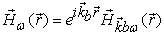(2)is the Bloch vector lying in the first Brillouin zone of the crystal’s reciprocal space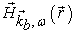and has the same periodicity as the underlying Bravais lattice. In considering the Eigenvalue problem for the frequency ω, Eq. (1), has a nonlinear character because the dielectric constant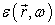, which acts as a scattering potential, it depends on the Eigenvalue ω. In terms of the electric fields, the solution of the differential Equation is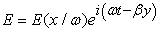Where E (x/ω) can be taken as the superposition of the incident and reflected wave in each medium, for jth layer can be written in the form(3)

whereand εj are the wave vector and dielectric constant in the jth layer respectively, and c is the speed of light in vacuum. The coefficients Aj and Bj have to be determined from the boundary condition that both electric field and its first derivative are continuous across an interface. The magnetic field vector along y-axis can be obtained by:(4)

Consider for the dielectric layer with no external current source and induced charge, the Maxwell equation becomes:and(5)

By using the Snell’s law in the above equation, the dielectric constant of the given materials is given by the following expressionOr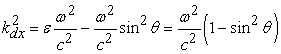or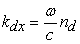(6)

Where the refractive index of the taken system is given by(7)

According to this theory, the transfer matrix methods are used to set the corresponding matrix of one period, which is given by the following expression:(8)

Whereand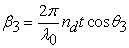,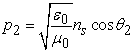,for TE mode and for TM-mode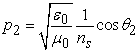,andis the wavelength in free space. The angle θ1 and θ2 determined by the Snell’s law of refraction are the ray angle in layer2 and 3 respectively. By using the above matrix, we try to construct the matrix for n-periods,(9)

Whereand UN are the Chebyshev polynomials of the second kind defined by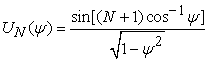(10)

By using the above relation, the matrix elements are given by the following formula;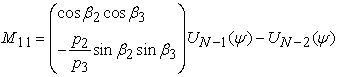(11)

The reflection and transmittance of the multilayered structure can be determined from the above Eq(16) and given as followsAnd(12)

Here p0 and ps are the first and the last medium of the considered structure and given by,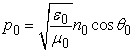and for TE-mode and for TM-mode,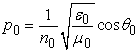,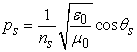. Both the media are taken to be a free space. The reflectivity and transmittance are given by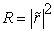and.

### 3. Numerical Results and Discussion

The rhombohedrally-distorted perovskite BiFeO3 has attracted much more attention because of ferroelectric behavior below 1100 K due to Bi displacement and as a G-type antiferromagnet below 640 K. The optical response in terms of the band gap of rhombohedrally-distorted perovskite BiFeO3 is observed around 2.7 eV with direct band gap and can be attributed to majority channel of Fe 3d→O 2p for charge transfer excitations. On the other hand, BiMnO3 is ferromagnetic below 105 K with a large saturation magnetization (3.6 μB per formula unit) and also shows the strong magneto-electric coupling. The optical absorption in BiMnO3 is ~0.75 eV (1650 nm), much lower than the ~2.7 eV (560 nm) in BiFeO3 and their thin ﬁlm grown by reactive molecular-beam epitaxy in an adsorption-controlled regime on buffered-HF treated (001) SrTiO3 and (110) DyScO3 substrates. Thus, these (0001) oriented ﬁlms have nearly single crystalline perfection, with three well-deﬁned crystallographicandaxes within the ﬁlm plane, and theaxis normal to the plane. The three y-z mirror planes in the 3m point group symmetry for BFO are thus well-deﬁned and give us to precisely linear and nonlinear optical coefficients. Optical spectra in transmittance mode were used to determine the optical band gap thin film materials. Corresponding substrates were used as references. Absorption was calculated as, where d is the film thickness, T is the measured transmittance, and E is the energy. The band gap of the materials is determined from the optical absorption curve. By utilizing the following relationship, the refractive index of the thin film is directly calculated:. The layer two is taken to be the dielectric layer (SrTiO3 or DyScO3) with a refractive index n2 = 2:437 or 4.58 and the thickness of the dielectric is taken to be ds = 90 nm, the selected number of periods is taken to be N = 10 and we also suppose that the electromagnetic wave normally incident on the interface. Considered in this structure, the different types of Multiferroic materials either BFO/BMO or substituted BFO with Mn addition is deposited on a dielectric substrate of thickness dM, the reflection spectra of the bilayer of periods N=10, can be obtained by using the matrix method in a stratified medium. According to the matrix methods, calculated either from TE methods or TM methods, the characteristics photonic spectra of the binary layer with N periods was calculated from the equation (12).

3.1. Reflection Spectra of BiFeO3 and BiMnO3 Multiferroic Material
3.1.1. Reflection Spectra of BFO with Dielectric

Before going to discuss the angle-dependent reflectance response, let us investigate the factors such as the number of periods, N, with the applied frequency of the incident wave. Two different materials i.e.; (001) plane of SrTiO3 and (110) plane DyScO3 were chosen as a dielectric for evaluating the reflection of the multilayer PC along with BFO/BMO. Dielectric constants K in the range of 18–35.5 and large optical band gaps are reported for single crystal SmScO3,GdScO3,DyScO3, and NdScO3, and epitaxial ﬁlms of LaScO3, GdScO3, and DyScO3 (with K~26, 20, and 22, respectively) were recently grown onto SrRuO3 /SrTiO3 substrates. Large optical band gaps and K values of ~22 were also observed on amorphous La-, Gd-, and Dy-scandate ﬁlms. The dielectric function of BiFeO3 is explained by using the Tauc–Lorentz oscillators sharing a common band gap and a constant additive term to consist of four ε1 denoted by ε(equal to 1 for this model). It consists of four Tauc–Lorentz oscillators which include oscillator amplitude A, broadening parameter Γ, resonance energy E0, and a Tauc gap Eg common to all oscillators [17, 18].

The first optical absorption occurs at 2.7 eV and the other absorption Tauc gap at 2.15 eV (577 nm) represents the onset of absorption, not the indirect gap but represents the direct optical band gap. The refractive index of the dielectric materials are 2.1 and 4.58 and their difference with BFO is around 0.383 with (001) plane of SrTiO3 and large of 2.097 with (110) plane of DyScO3. The density of the spectra depends upon the number of periods considered in the photonic structure . Their results show that as we increase the number of layers the reflection band becomes wider and wider for the same value of refractive index contrast and for the same thickness which is shown in the Figure 2. To increase the number of periods, the density of the spectra is much more concentrated within same the wavelength region, shown in Figure 2 (a) and Figure 2 (b). The intensity of the spectra increases with increase the number of the periods along with a shift in wavelength towards the left side, which is tabulated in Table 1.

#### Table 1. Wavelength position & percentage reflection of BFO-PC

Figure 2. The calculated wavelength-dependent reflectance of a binary PC (a) combination of BFO and SrTiO3 (b) combination of BFO and DyScO3 at different N =2; 4; 6; 8, 10 and 15

At SrTiO3, 99.83 % reflection is obtained at N=15 but only 27.1 % reflection is maintained at N=2 whereas DyScO3 dielectric gives 99.62% reflection at N=15 layer while 35.6 % reflection arises from N=2 layer. From N=2 To N=4, the intensity of the reflection increase 2.3 times while 1.33 to 1.01 times the intensity of the reflection spectra were observed to increase the periods from N=4 to N=15. It is clearly seen that there exists a PBG with a bandwidth of 122.4 nm because its left and right band edges are λL = 431.54 nm and λR = 553.94 nm in SrTiO3 and bandwidth in DyScO3 is obtained around 183.687 nm with left and right band edges at λL = 780.346 nm and λR = 964.033 nm. Instead of one bandwidth in the infrared region with DyScO3 dielectric, more two pass band rises in the visible region with small widen. To change the dielectric from SrTiO3 to DyScO3, the left and right band edges of the maximum intensity peak shifts from the visible region to the infrared region with near about same bandwidth. But one of the pass present in the visible region but the position of the other pass band does not change much more with the change the nature of the dielectric. It is also evident from the Figure 2 that the flattop PBW can be reached as N = 15. To compare the results with N = 2 in Figure 2 (a) and Figure 2(b), we see that the large difference only appears in the side lobes. That is, the value of N is at least taken to be 15 for obtaining better flatness in the spectral reflection. BFO also shows the optical absorption band near about 2.15 eV (direct band gap).

Figure 3 shows the calculated wavelength-dependent reflectance of a binary PC of BFO with SrTiO3 in the different optical absorption region. It is also seen that the band gap in Figure 3 can be enhanced due to the increase in the index contrast n1 by n2 being fixed. It is evident from Figure 3, that there exists a PBG with a bandwidth of 122.4 nm because its left and right band edges are λL = 431.54 nm and λR = 553.94 nm in I-optical region, but it is slightly shifted towards the higher wavelength sides with a bandwidth of 139.13 of slightly shifts of the right band edges from λR = 553.94 nm to λR = 570.67 nm in II-optical region. The variation in the index contrast in the dielectric not only affects the band gap extension, but also shift in the band gap from lower wavelength to the higher wavelength sides.

Reflectance spectra of multilayer structure of BFO on the substrate at various angles of incidents are also plotted in Figure 4 (a) & Figure 4 (b). We can see clearly from Figure 4 that there exists OBG at the different angle of incident obviously. The frequency range of OBG runs from 446.07 to 525.08 nm, and the bandwidth is 79.01nm at 10° angle of incident as shown in Figure 4 (a) at (001) plane of the SrTiO3 substrate.

The OBG is obviously enlarged when the angle of incidence increases, as compared to the normal-incidence OBG in Figure 4 (a). To increase the angle of incident from 10 to 83o, the left and right band edges are shifted towards the lower wavelength with slightly enlarge OBG bandwidth. SrTiO3 and DyScO3 with large difference in refractive index shows the same corresponding results at the different angle of incident and their results are tabulated in Table 2.

#### Table 2. Position & width of OBG of BFO multilayer structure

Figure 4. Reflectance spectra of 1D-PC of BFO and (a) SrTiO3-substrate (b) DyScO3-substrate at various incident angles and their spectra are calculated by TMM

It also can be seen that the lower and upper band edges of the OBG are more sensitive to the increase of the angle of incident for both TE and TM-polarizations. With change the dielectric materials from SrTiO3 to DyScO3, that there is a large shift in the band edges from the lower wavelength to the higher wavelength, with a slightly higher bandwidth of BFO-PC, as shown in Figure 4 (a) and Figure 4 (b) at 10° angle of the incident, but at the other angle of the incident, the shift of band edges and bandwidth is not enlarged as much to change the incident wavelength. From 30 to 89° angle of an incident, there is no change in band edges and the bandwidth of the BFO-PC. Thus, from the analysis of the above results, TE omnidirectional gap determines the bandwidths of the OBG.

3.1.2. Reflection Spectra of BMO with Dielectric (001) Plane of SrTiO3 and (110) Plane of DyScO3

Next let us discuss the reflection spectra of multilayer structure of BMO with (001) plane of SrTiO3 and (110) plane of DyScO3. In this case, the first optical absorption occurs at ~0.75eV much lower than the ~2.2 eV, (560 nm) onset in BiFeO3 thin films. The peaks occur at ~1.6 eV (780 nm) and ~5.5 eV (230 nm), with a broad shoulder near 4 eV. This can be explained by the following reasons for the 1.6 eV near infrared excitation in BiMnO3: (i) on-site excitations between crystal field split 3d levels, (ii) charge transfer excitations involving strongly hybridized Mn3+ and oxygen states, or (iii) transitions between localized Hubbard bands. By using these band gaps, the refractive index of the BMO at (001) plane of SrTiO3 and (110) plane of DyScO3 is directly calculated from optical band gap with three distinct values of the refractive index in the different optical region. The band gap of the materials is determined by majority carriers, and minority states are involved only above 3 eV. Higher energy excitations [above 3.7 eV (330 nm)] are assigned as O 2p→ Mn 3d charge transfer, with some mixing of Bi 6p levels above 3 eV. This excitations actually change the band-gap of the existing materials and also changes the reflectance spectra of the multilayer materials designated alternatively with either (001) plane of SrTiO3 and (110) plane of DyScO3 as a dielectric material.

Figure 5 shows the reflectance spectra of the BMO-PC at the different dielectric materials with the angle of incidence 30o. The results obtained from the spectral analysis are also presented in Table 3. The OBF is to be enlarged to increase the refractive index of the BMO, in the different optical region. At n=3. 7, BMO-PC shows enlarged band gap of 436.88 and the position of λL and λR are shifted from the lower wavelength region to higher wavelength region, as shown in Figure 5 (a). While at n=1. 93, the OBG are found in the UV-region with small band gap width. It is also clearly evident from the Figure 5 that the reflectance spectra of BMO on SrTiO3 slightly shifted towards the lower wavelength sides with an enlarge band gap as compared to DyScO3 with increase the refractive index from n=1. 93 to n=3.7.

Figure 5. Reflectance spectra of 1D-PC of BMO at (a) SrTiO3-substrate (b) DyScO3-substrate at various refractive index and their spectra are calculated by TMM (angle taken in this case 30o)

Figure 6 shows the variation of the reflectance spectra of 1D-PC of BMO with SrTiO3 as a dielectric material. For the calculation of reflectance the refractive index is taken to be 1.93. To increase the number of periods, the reflectance spectra are much more concentrated within the same wavelength region without changing the width of the optical band gap. The largest change is only observed at the small number of periods while the width of the optical band gap is not altered by varying the number of periods from N=4 to N=15, as shown in Figure 6. The left and right position of the band edges and their width of the BMO-PC are calculated by using the TMM and their results are tabulated in Table 3 with respect to increasing order of the periods.

Figure 6. The calculated wavelength-dependent reflectance of a binary PC, having a combination of BMO and SrTiO3 at different N =2; 4; 6; 8, 10 and 15

#### Table 3. Position & width of OBG of BFO multilayer structure

The number of periods just increases the density of the spectra but the position of the left and right is changed by changing the refractive index of the existing materials in the different optical region which is shown in Figure 7. Figure 8 shows the variation of the optical band gap of 1D-PC of BMO with the different dielectric materials with respect to the number of periods.

Figure 7. Shift in position of the left and right band edges of binary photonic crystals of (A) BMO and SrTiO3 (B) combination of BFO and DyScO3 with the number of the periods
Figure 8. Optical Band gap of binary photonic crystals of (A) BMO and SrTiO3 (B) combination of BFO and DyScO3 with the number of the periods

BMO with SrTiO3 shows higher optical band gap with the variation of refractive index of BMO in the different optical region as compared to DyScO3 as a dielectric material as shown in Figure 8.

3.1.3. Tunable Band Gap in Bi (Fe1−xMnxO3) Films on SrTiO3 (001) Fubstrates

To calculate the reflectance spectra from TMM methods our investigation based on the theoretical results were carried out on a series of 33 and 50 nm thick Bi (Fe1−xMnxO3) films grown by reactive molecular-beam epitaxy in an adsorption-controlled regime on SrTiO3 (001) substrates . The measurements of the optical spectra, collected in transmittance mode using a Perkin–Elmer Lambda-900 spectrometer (3000–190 nm; 0.41–6.53 eV), reveal an absorption band at 1.6 eV that develops with increasing Mn concentration. Figure 9 shows the 300 K optical absorption spectrum of a series of Bi (Fe1−xMnxO3) solid solution films including the two end members BiFeO3 and BiMnO3 (calculation is based on the phase diagram BiFeO3 and BiMnO3). The unit cell of BiFeO3 can be considered to be a deformed ideal cubic perovskite. By moving Bi and Fe atoms along the pseudocubic  direction and O atoms along the pseudocubic  directions, the unit cell is elongated with the neighboring octahedrons rotated in different directions about the  axis shows the maximum optical absorption at 2.7eV, as shown in Figure 9. It is preceded by a small shoulder, centered at ~2.5 eV, which yields an absorption onset near 2.2 eV. Peaks at 3.2 and 4.5 eV are due to dipole-allowed Fe 3d→O 2p for charge transfer excitations. At the other end (when Fe is completely replaced by Mn), due to the Bi 6s2 lone pairs, the unit cell of the BiMnO3 bulk crystal is distorted to C2 symmetry at and below room temperature shows the optical absorption in BiMnO3 at ~0.75 eV (1650 nm) which is much lower than the ~2.2 eV (560 nm) onset in BiFeO3 thin films. The peaks occur at ~1.6 eV (780 nm) and ~5.5 eV (230 nm), with a broad shoulder near center 4 eV, which caused by Fe3+ 6A14T1 and 6A14T2 crystal-field transitions, which will result in an underestimation of the extinction coefficient around these wavelengths.

Figure 9. Absorption spectrum of Bi (Fe1−xMnxO3) thin films along with BiFeO3 and BiMnO3 at 300 K on SrTiO3, substrate where x=0, 0.2, 0.4, 0.6, 0.8, and 1

Figure 10 shows the variation of band gap and refractive index with the percentage of Mn in the host lattice. But from moving BiFeO3 to BiMnO3, by incorporating Mn into the BiFeO3 shows the expansion of the lattice which enhanced the magnetization. With increasing Mn substitution, the 2.7 eV charge gap broadens and begins to red shift, as shown in Figure 10. This trend continues in Bi (Fe0.8Mn0.2) O3 and Bi (Fe0.6Mn0.4) O3. The 2.5 eV shoulder is rarely visible in Bi (Fe0.8Mn0.2) O3, and it disappears in films with higher Mn3+ concentrations. At the same time, a new structure appears in the near infrared, grows with increasing Mn3+ substitution in the host lattice of BFO, and eventually develops into the 1.6 eV band in BiMnO3. At the same time, a new structure appears which is very active in the near infrared, grows with increasing Mn3+ substitution in the host lattice, and eventually develops into the 1.6 eV band in BiMnO3.

Figure 10. Energy threshold extracted from absorption data at 300 K using the direct gap method (A) variation of Mn in BiFeO3 (B) variation of Fe in BiMnO3 (where examples of BiMnO3 and BiFeO3 are shown)

Figure 11 shows the reflectance spectra of 1D- binary PC of BFO, BMO and substituted with Mn+3 in BFO host lattice with SrTiO3 as a dielectric material at 30o angle of incidence. It can be seen in the Figure 11 that each and every layer of BFO substituted with the Mn+3 presents the distinct band gap in the certain interval of wavelength. This interval of wavelength is defined in terms of left and right band edges and their results are tabulated in Table 4. Form Table 4, it is also observed that the left band edges and right band edges don’t show much more variation with the substitution of Mn+3 either from the BFO sides or BMO sides. Not only band gap, but also the refractive index of the materials depends upon the percentage content of the doping elements and the sites where it sits in the lattice. By the substitution of Mn+3 in the BFO, Mn+3 occupies +B sites in the perovskites structure in which two different B-site cations form a super-lattice within the ABO3 perovskite structure.

#### Table 4. Position & width of OBG of BFO multilayer structure

Due to the large orbital radius of the Bi 6s2 lone pairs, the crystal structure of bismuth-based perovskites is usually distorted to lower symmetries, producing large spontaneous ferroelectric polarization along certain crystallographic directions. By the substitution of Mn+3 in the host lattice, the host lattice is deformed largely due to development of stain in the lattice because of dissimilar radii of substituted doping elements on the +B sites in perovskites structure. For Fe3+(3d5), the five spins are aligned in a half-filled 3d shell (t2g3 eg2) high-spin configuration as dictated by the Hund’s rule; for the same reason, Mn3+ (3d4) is in a high-spin t2g3 eg1 arrangement in octahedral B sites that is further stabilized by a strong Jahn-Teller axial distortion along the z axis. An important characteristic of the perovskite lattice is the 180-degree cation-anion-cation bonds that involve the eg orbital’s in a bonding/antibonding linkages with the oxygen 2p orbital’s, while relegating the t2g orbital to nonbonding roles. Jahn-Teller axial distortion along the z axis is again distorted by the substitution of doping element and also caused the z-axis maximum elongated. This factor is very important and responsible criteria for changing the band gap and refractive index of the existing materials with the addition of doping elements. Table 4 represents the change in the band edges with the addition of the Mn+3 in the lattice due to changes in the band gap of the existing materials.

Figure 12. Change in bandwidth of 1D-PC of BFO, BMO and substituted with Mn+3 multilayer structure with SrTiO3-substrate (where N=15 and incident angle =30o)

Figure 12 shows the variation of bandwidth with the percentage of Mn+3 in the lattice. Mn+3 substituted in BFO shows the small band gap at the lower wavelength sides whereas, the red shift is observed in BMO substituted by Fe+3. Figure 13 shows reflectance spectra of the BFO substituted with Mn+3, but in this case the thickness of the Bi (Fe1−xMnxO3) is changed from 33 nm to 50nm.

By the substitution of Mn+3 from the BFO sides, the right and left band edges are observed at 434.36 and 151.38 nm along with the bandwidth of 282.98nm while the red shift is observed with change the thickness from 33 nm to 50nm and the right and left band edges are found at 240.80 and 543.48 with enhanced bandwidth of 302.68. But from the other end, substitution of Mn+3 from BMO sides affects the width of band gap and the position of left and right band edges and it is observed from the Fig.13, that bandwidth is largely enhanced to increase the thickness from 33 to 50nm from BMO sides. At 33 nm the position of the left and right band edges are at 201.09 and 568.15nm with a bandwidth of 367.05nm but the position of left and right band edges are changed to change the thickness of the layered materials and it is located at 275.50, and 712.37nm and their width is 436.87nm.

Figure 13. Optical Band gap of binary photonic crystals of (A) BMO and SrTiO3 (B) combination of BFO and DyScO3 with the thickness of the growing film

### 4. Conclusion

In conclusion, we measured the optical properties of Bi (Fe1−xMnx) O3 solid solution films on the different substrate and show that thin film of the polar oxides like the mixed metal system studied here have the potential to collect light in the near infrared range of the solar spectrum, separate charge by virtue of the intrinsic ferroelectricity character of the used materials. The reflectance spectra of the PC crystals depend upon the substitution of the Mn+3 at the place of Fe+3 which form the solid solution with host materials. Due to the formation of the solid solution, the ferroelectric materials are able to absorb the electromagnetic radiation in the region of the electromagnetic spectrum which caused the change in bandgap of the existing materials. This is due to charge transfer processes from Fe+3 to Mn+3; connect very efficiently with other important energy levels in a working solar device. The tunability in the material was observed by either change the refractive index or the thickness of the growing film. The numbers of the periods also enhance the density of the reflectance spectra of the PC. The tenability arises due to absorption of electromagnetic light in different optical region. With increase the content of Mn+3 in BFO, not only a bandgap but also affects the refractive index of the dielectric materials. The band gap tuning in the ferroelectric materials may be present in other mixed metal polar oxides which forms easily solid solution.

### Reference

  Yablonovitch, E., “Inhibited spontaneous emission in solid-state physics and electronics,” Phys. Rev. Lett., Vol. 58, 2059, 1987.In article  John, S., “Strong localization of photons in certain disordered dielectric superlattices,” Phys. Rev. Lett., Vol. 58, 2486, 1987.In article  Suthar, B. and A. Bhargava, “Tunable multi-channel filtering using 1-D photonic quantum well structures,” Progress In Electromagnetics Research Letters, Vol. 27, 43-51, 2011.In article CrossRef  Bhargava, A. and B. Suthar, “Optical switching properties of kerr- nonlinear chalcogenide photonic crystal,” J. of Ovonic Research, Vol. 5, No. 6, 187, 2009.In article  Li, B., Zhou J., Li L., Wang X. J., Liu X. H., and Zi J., “Ferroelectric inverse opals with electrically tunable photonic band gap,” Appl. Phys. Lett., Vol. 83, 4704, 2003.In article  Kumar, V., Singh K. S., and Ojha S. P., “Broadening of omnidirectional photonic band gap in Si-based one-dimensional photonic crystals,” Progress In Electromagnetics Research M, Vol. 14, 101-111, 2010.In article CrossRef  Wang, X., Kempa K., Ren Z. F., and Kimball B., “Rapid photon flux switching in two-dimensional photonic crystals,” Appl. Phys. Lett., Vol. 84, 1817, 2004.In article CrossRef  Fink, Y., Winn J. N., Fan S., Chen C., Michel J., Joannopoulos J. D., and Thomas E. L., “A dielectric omnidirectional reflector,” Science, Vol. 282, 1679-1682, 1998.In article CrossRef  Chigrin, D. N., Lavrinenko A. V., Yarotsky D. A., and Gaponenko S. V., “Observation of total omnidirectional reflection from a one-dimensional dielectric lattice,” Appl. Phys. A: Mater.Sci. Process. Vol. 68, 25-28, 1999.In article CrossRef  Yablonovitch, E., “Photonic band-gap structures,” J. Opt. Soc. Amer. B, Vol. 10, 283-295, 1993.In article CrossRef  Li, H. H., “Refractive index of silicon and germanium and its wavelength and temperature derivatives,” J. Phys. Chem. Ref. Data, Vol. 9, 561, 1980.In article  Xu X. S., Ihlefeld J. F., Lee J. H., Ezekoye O. K., Vlahos E., Ramesh R., Gopalan V., Pan X. Q., Schlom D. G., and Musfeldt J. L., “Tunable band gap in Bi (Fe1−xMnx)O3 films” Applied Physics Letters 96, 192901, 2010.In article CrossRef  Seema H., Durrani S. K., Saeed K., Mohammadzai I. and Hussain N. “Auto –Combustion synthesis and characterization of Multi-ferroic (BiFeO3) Materials” JPMS Conference Issue, Materials 2010In article  Carvalho T.T., Tavares P.B. “Synthesis and thermodynamic stability of multiferroic BiFeO3” Materials Letters 62, 3984-3986, 2008.In article CrossRef  Zhao Y. Miao J. Zhang X. Chen Y. Xu X. G. Jiang Y. “Ultra-thin BiFeO3 Nanowires prepared by a Sol–gel combustion method: an investigation of its multiferroic and optical properties” J Mater Sci: Mater Electron (2012) 23:180-184.In article CrossRef  Yeh, P., Optical Waves in Layered Media, John Wiley & Sons, Singapore, 1991.In article  Kumar Amit, Ram Rai C, Podraza Nikolas J., Denev Sava, Ramirez Mariola, Chu Ying-Hao, Martin Lane W., Ihlefeld,Jon Heeg T., Schubert J., Schlom Darrell G., Orenstein J., Ramesh R., Collins Robert W., Musfeldt, Janice L. and Gopalan V., “Linear and nonlinear optical properties of BiFeO3” Applied Physics Letters 92, 121915 (2008).In article  Lee J. H., Ke X., Misra R., Ihlefeld J. F., Xu X. S., Mei Z. G., Heeg T., Roeckerath M., Schubert J., Liu Z. K., Musfeldt J. L., Schiffer P., and Schlom D. G. “Adsorption-controlled growth of BiMnO3 films by molecular-beam epitaxy” Applied Physics Letters 96, 262905 (2010).In article CrossRef  Xu X. S., Ihlefeld J. F., Lee J. H., Ezekoye O. K., Vlahos E., Ramesh R., Gopalan V., Pan X. Q., Schlom D. G., and Musfeldt J. L., “Tunable band gap in Bi(Fe1−xMnx)O3 films” Applied Physics Letters 96, 192901 (2010).In article CrossRef  Lee, J. H. Ke X., Misra R., Ihlefeld J. F., Xu X. S., Mei Z. G., Heeg T., Roeckerath M., Schubert J., Liu Z. K., Musfeldt J. L., Schiffer P., and Schlom D. G. “Adsorption-controlled growth of BiMnO3 films by molecular-beam epitaxy” Applied Physics Letters 96, 262905 (2010).In article CrossRef  Xu X. S., Ihlefeld J. F., Lee J. H., Ezekoye O. K., Vlahos E., Ramesh R., Gopalan V., Pan X. Q., Schlom D. G., and Musfeldt J. L.. “Tunable band gap in Bi (Fe1−xMnxO3) films” Applied Physics Letters, 192901 (2010).In article CrossRef